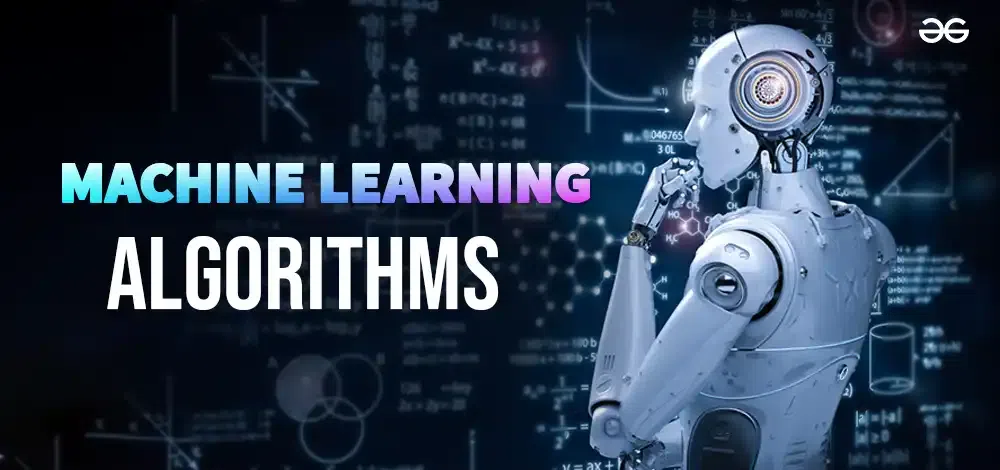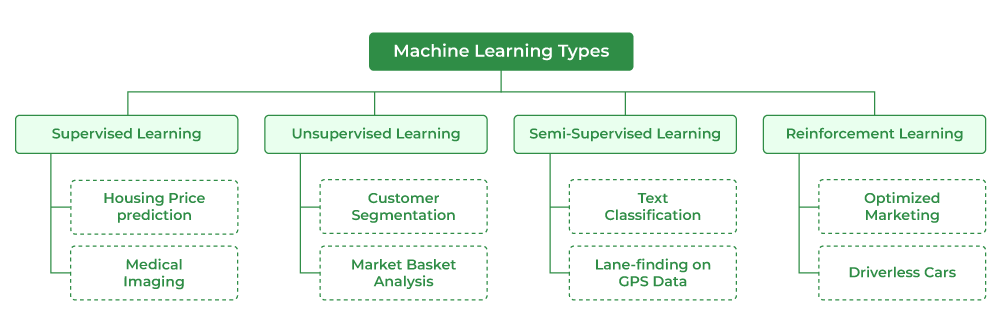# Machine Learning Algorithms

Machine learning algorithms are computational models that allow computers to understand patterns and forecast or make judgments based on data without the need for explicit programming. These algorithms form the foundation of modern artificial intelligence and are used in a wide range of applications, including image and speech recognition, natural language processing, recommendation systems, fraud detection, autonomous cars etc.This Machine learning Algorithms article will cover all the essential algorithms of machine learning like Support vector machine, decision-making, logistics regression, naive bayees classifier, random forest, k-mean clustering, reinforcement learning, vector, hierarchical clustering, xgboost, adaboost, logistics, etc.

## Types of Machine Learning Algorithms

There are three types of machine learning algorithms.

1. Supervised Learning
2. Unsupervised Learning
3. Reinforcement Learning## 1. Supervised Learning Algorithm

Supervised learning is a type of machine learning algorithms where we used labeled dataset to train the model or algorithms. The goal of the algorithm is to learn a mapping from the input data to the output labels, allowing it to make predictions or classifications on new, unseen data.

Supervised Machine Learning Algorithms

1. Linear Model:
2. K-Nearest Neighbors (KNN):
3. Support Vector Machines:
• Different Loss functions in SGD
5. Decision Tree:
6. Ensemble Learning:
7. Generative Model
8. Time Series Forecasting:
9. Supervised Dimensionality Reduction Technique:
• Linear Discriminant Analysis (LDA)

Some of the Supervised Machine Learning Algorithms can be used used for both Classification & Regression with a little bit of modification

## 2. Unsupervised Learning Algorithm

Unsupervised Learning is a type of machine learning algorithms where the algorithms are used to find the patterns, structure or relationship within a dataset using unlabled dataset. It explores the data’s inherent structure without predefined categories or labels.

Unsupervised Machine Learning Algorithms

## 3. Reinforcement Learning

Reinforcement Learning is a type of machine learning algorithms where an agent learns to make successive decisions by interacting with its surroundings. The agent receives the feedback in the form of incentives or punishments based on its actions. The agent’s purpose is to discover optimal tactics that maximize cumulative rewards over time through trial and error. Reinforcement learning is frequently employed in scenarios in which the agent must learn how to navigate an environment, play games, manage robots, or make judgments in uncertain situations.

Reinforcement Learning

## List of Popular Machine Learning Algorithm

Here is a list of the Top 10 Most popular Machine Learning Algorithms.

### 1. Linear Regression

Linear regression is a simple algorithm used to map the linear relationship between input features and a continuous target variable. It works by fitting a line to the data and then using the line to predict new values.

### 2. Logistic Regression

Logistic regression is an extension of linear regression that is used for classification tasks to estimate the likelihood that an instance belongs to a specific class.

### 3. SVM (Support Vector Machine)

SVMs are supervised learning algorithms that can perform classification and regression tasks. It finds a hyperplane that best separates classes in feature space.

### 4. KNN (K-nearest Neighbour)

KNN is a non-parametric technique that can be used for classification as well as regression. It works by identifying the k most similar data points to a new data point and then predicting the label of the new data point using the labels of those data points.

### 5. Decision Tree

Decision trees are a type of supervised learning technique that can be used for classification as well as regression. It operates by segmenting the data into smaller and smaller groups until each group can be classified or predicted with high degree of accuracy.

### 6. Random Forest

Random forests are a type of ensemble learning method that employs a set of decision trees to make predictions by aggregating predictions from individual trees. It improves the precision and resilience of single decision trees. It can be used for both classification and regression tasks.

### 7. Naive Bayes

Naive Bayes is a probabilistic classifier based on Bayes’ theorem that is used for classification tasks. It works by assuming that the features of a data point are independent of each other.

### 8. PCA (Principal Component Analysis)

PCA is a dimensionality reduction technique used to transform data into a lower-dimensional space while retaining as much variance as possible. It works by finding the directions in the data that contain the most variation, and then projecting the data onto those directions.

### 9. Apriori algorithms

Apriori algorithm is a traditional data mining technique for  association rules mining in transactional databases or datasets. It is designed to uncover links and patterns between things that regularly co-occur in transactions. Apriori detects frequent itemsets, which are groups of items that appear together in transactions with a given minimum support level.

### 10. K-Means Clustering

K-Means clustering is an unsupervised learning approach that can be used to group together data points. It works by finding k clusters in the data so that the data points in each cluster are as similar to each other as feasible while remaining as distinct as possible from the data points in other clusters.

Discover the fundamental concepts driving machine learning by learning the top 10 algorithms, such as linear regression, decision trees, and neural networks.

## Machine Learning Algorithm – FAQs

### 1. What is an algorithm in Machine Learning?

Machine learning algorithms are techniques based on statistical concepts that enable computers to learn from data, discover patterns, make predictions, or complete tasks without the need for explicit programming. These algorithms are broadly classified into the three types, i.e supervised learning, unsupervised learning, and reinforcement learning.

### 2. What are types of Machine Learning?

There are mainly three types of machine learning:

• Supervised Algorithm
• Unsupervised Algorithm
• Reinforcement Algorithm

### 3. Which ML algorithm is best for prediction?

The ideal machine learning method for prediction is determined by a number of criteria, including the nature of the problem, the type of data, and the unique requirements. Support Vector Machines, Random Forests, and Gradient Boosting approaches are popular for prediction workloads. The selection of an algorithm, on the other hand, should be based on testing and evaluation of the specific problem and dataset at hand.

### 4. What‌ ‌are‌ ‌the‌ ‌10 ‌Popular‌ ‌Machine‌ ‌Learning Algorithms?‌

Below is the list of Top 10 commonly used Machine Learning (ML) Algorithms:

1. Linear Regression
2. Logistic Regression
3. SVM (Support Vector Machine)
4. KNN (K-nearest Neighbour)
5. Decision Tree
6. Random Forest
7. Naive Bayes
8. PCA (Principal Component Analysis)
9. Apriori algorithms
10. K-Means Clustering

Whether you're preparing for your first job interview or aiming to upskill in this ever-evolving tech landscape, GeeksforGeeks Courses are your key to success. We provide top-quality content at affordable prices, all geared towards accelerating your growth in a time-bound manner. Join the millions we've already empowered, and we're here to do the same for you. Don't miss out - check it out now!